Home Data Structures Basics: Bits & Bytes Bitwise Manipulation Tutorial

# Bitwise Manipulation Tutorial

Bitwise manipulation is a technique to modify a bit of a number to get the desired result. It’s a powerful technique to optimize and speed up your code. Bitwise manipulation proves very useful in coding interviews and competitive programming.

The prerequisites for bitwise manipulation is understanding of binary number system read here…

### The Basics

Before jumping into the complex bitwise manipulation operations lets start by revising or learning the basics.

###### 1) AND ( & )

AND operator compares each bit a particular position of both the given numbers and sets it to 1 if both are one or 0 if at least one of them 0.

```5 & 13 = 5
5 = 01012
13 = 11012

5 & 13 =  0 1 0 1
& 1 1 0 1
----------
0 1 0 1  (01012 = 5)
```
###### 2) OR ( | )

The OR operator compares the bits of two numbers and sets it to one if either of the bit is 1.

```5 | 13 = 13
5 = 01012
13 = 11012

5 & 13 =   0 1 0 1
| 1 1 0 1
----------
1 1 0 1 (11012 = 13)```
###### 3) Bitwise Complement ( ~ )

The bitwise complement of any number N is -(N+1)

`~ 5 = -16`
###### 4) XOR ( ^ )

The XOR operator sets the resulting bit to 1 if both the bits(being compared) are different else sets the resulting bit to 0.

```5 ^ 13 = 8
5 = 01012
13 = 11012
5 ^ 13 =   0 1 0 1
^ 1 1 0 1
----------
1 0 0 0 (10002 = 8)```
###### 5) XNOR ( ~ ^ )

The XNOR operator works the opposite of XOR. It sets the resulting bit to 0 if both the bits(being compared) are different else sets the resulting bit 1. There is no predefined boolean operator for XNOR. It’s a combination of XOR and NOT

```5 XNOR 13  or ~(5^13) = 7
5 = 01012 13 = 11012

5 ^ 13 = 0 1 0 1 ^ 1 1 0 1 = 1 0 0 0

~(5 ^ 13) = ~(1 0 0 0) =  0 1 1 12 (01112 = 7)```

Here is the truth table of all the major operation on a single bit:

 A B A & B A | B ~ A A ^ B 0 0 0 0 1 0 0 1 0 1 1 1 1 0 0 1 0 1 1 1 1 1 1 0

### Arithmetic & Logical Shift

###### 1) Arithmetic Right Shift ( >> )

It is indicated with >> operator in any programming language.

Arithmetic Right shift we shift the value to the right and fill it with the sign of the bits(or the MSB). Since the positive number is represented with 0 in the most significant bit(MSB) and the negative number is represented with the most significant bit(MSB) as 1, so right sift of +ve number will fill the new bits with 0 and in case of the negative number, we will fill the new bits with 1.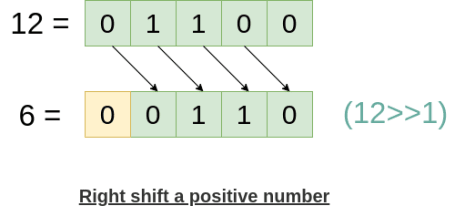In 1bit arithmetic right, the number is shifted right by 1 bit and the least significant bit is lost, while the most significant bit is filled with 0, because it’s a positive number.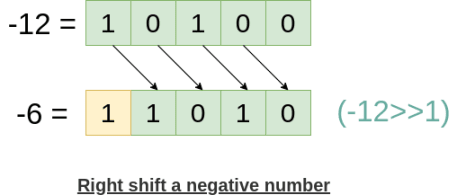In 1bit arithmetic right, the negative number is shifted right by 1 bit, the least significant bit is lost, while the most significant bit is filled with 1 because it’s a negative number.

If we look carefully we will find, in the arithmetic right shift, we are (roughly) dividing a number(either positive or negative) by 2.

###### 2) Arithmetic Left Shift ( << )

It is indicated with << operator in any programming language.

Arithmetic Left shift we shift the value to the right and fill the new bits with 0 for both positive and negative numbers.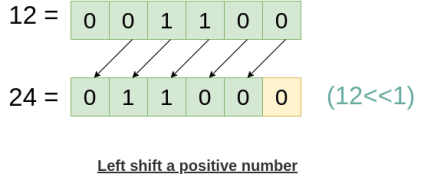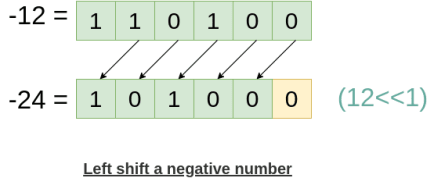Notes:

1. The arithmetic left shift is equivalent to multiplying a number by 2.
2. The arithmetic left to shift for negative numbers will result in negative numbers only until there is an integer overflow i.e, we are left shifting beyond the range of integer.
###### 3) Logical Right Shift ( >>> )

It is indicated with >>> operator, but this operator is not explicitly present every programming language.

The logical right shift the bits to the right and put 0 in the most significant bit even if the number is negative with MSB as 1.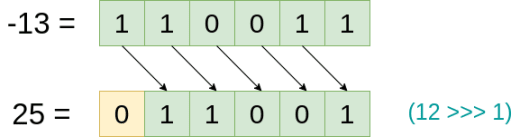Here we have assumed the size of integer 6-bit, hence we got the output as 25 but in real-time(actual computer) the size of the integer is 4B ≈ 32 bit and you will get a different result. But the logic is going to remain the same.

Following is the result in node js, with the size of int 4B:  -13>>>1 = 2147483641

### Get, Set, Clear and Update a Single Bit

###### 1) Get Bit

The Get bit method helps us to find whether the bit at position i is 0 or 1 where i is any random position.

It works by the first shifting 1 left by i bits (1<<i) bits then performing AND with the actual number (num & (1<<i)) and finally compared with zero to check if the ith bit 0 or 1.

```num = 29, check if 4th and 1st bit is 1 or 0

num = 29 ≈ 0111012

i = 4 and 1<<i = 1<<4 = 16 (0100002)
mask = num & (1<<4) = 29 & 16 = 16

29 & 16 =    0 1 1 1 0 12
& 0 1 0 0 0 02
----------------
0 1 0 0 0 0    (0100002 = 16)

Now, mask!=0, then means 4th bit is 1.

i = 1 and 1<<i = 1<<1 = 2 (0000102)
mask = num & (1<<2) = 29 & 2 = 0

29 & 16 =   0 1 1 1 0 12
& 0 0 0 0 1 02
---------------
0 0 0 0 0 0 (0000002 = 0)

Now, mask == 0, hence the 2nd bit is 0```

Here is the algorithm to Get bit at ith position:

```Get_bit(num, i){
}```
###### 2) Set Bit

Set bit method sets the bit at position i to 1, where i is any random position.

It works by the first shifting 1 left by i bits (1<<i) bits then performing OR with the actual number (num & (1<<i)).

Here is the code to set a bit at the ith position to 1.

```Set_bit(num, i){
}```
###### 3) Clear Bit

Clear bit method helps us to set the bit at position i to 0, where i is any random position.

It works by the first shifting 1 left by i bits (1<<i) bits then taking the complement of it or negating it ~(1<<i) and finally performing AND with the actual number (num & (1<<i)).

```num = 29, clear the 4th bit

num = 29 ≈ 0111012

i = 4 and 1<<i = 1<<4 = 16 (0100002)
~(i<<4) = ~(010000) = (101111)
mask = num & ~(1<<4) = 29 & 47 = 13

29 & 47 =   0 1 1 1 0 12
& 1 0 1 1 1 12         (we have assumed 6 bit integer)
----------------
0 0 1 1 0 1 (0011012 = 13)

The 4th bit is successfully cleared```

Here is the code to clear a bit at the ith position (making ith bit 0).

```Clear_bit(num, i){
}```
###### 4) Update Bit

The update bit method allows us to update the bit at position i to 0 or 1, where i is any random position.

For updating the ith bit, we will first clear the ith bit using the above (clear bit) method and then set the bit at the ith position to s(s can be 0 or 1)

```num = 29, update the 1st bit to 1

num = 29 ≈ 0111012
s = 1

i = 2 and 1<<i = 1<<2 = 2 (0000102)
~(i<<2) = ~(000010) = (111101)
mask = num & ~(1<<2) = 29 & 61 = 29

29 & 47 =  0 1 1 1 0 12
& 1 1 1 1 0 12      (we have assumed 6 bit integer)
----------------
0 1 1 1 0 1  (0111012 = 29)

The 2th bit is successfully cleared, now setting it to s.

29 | (s<<1) = 29 | 2 = 31

29 | 2 =   0 1 1 1 0 12
| 0 0 0 0 1 02
---------------
0 1 1 1 1 12  = 31
```

Following is the code to update a bit at the position i to s (making ith bit s).

```Update_bit(num, i, s){